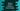# 2 ways to check if a number is Pronic number or not in Python## Python program to check if a number is a Pronic number or not:

In this post, we will learn how to check if a number is a Pronic number or not in Python. We will learn how to find if a number is a Pronic number or not and how to use that method to check for different numbers.

Before we start writing the program, let’s understand what is a Pronic number and how to write a program that checks for Pronic number from a user input value.

### What is a Pronic number:

A number is called a Pronic number if we can represent it as a product of two consecutive numbers. For example, 12 is a Pronic number, because we can represent it as 3 * ( 3 + 1). For any number that can be represented as n * (n + 1), that will be a Pronic number.

### Method 1: Find if a number is a Pronic number or not by using a loop:

In this method, we will use a loop starting from 1 to the number. For each value in that loop, it will check num * (num + 1), and if the value is equal to that number itself, it will be a Pronic number.

Let’s write down the program:

``````def check_pronic(no):
for i in range(no):
if i * (i + 1) == no:
return True
if i * (i + 1) > no:
return False

given_num = int(input('Enter a number: '))

if check_pronic(given_num):
print(f'{given_num} is a Pronic number')
else:
print(f'{given_num} is not a Pronic number')``````

Here,

• check_pronic method is used to check if a number is Pronic or not. It takes one number as the parameter and returns one Boolean value, True if the number is Pronic, else it returns False.
• This program is taking the number as input from the user and storing that value in given_num.
• This number is passed to check_pronic and based on its return value, we are printing a message.

If you run this program, it will print output as like below:

``````Enter a number: 20
20 is a Pronic number

Enter a number: 21
21 is not a Pronic number``````

### Method 2: Find if a number is a Pronic number or not by using square-root:

Another way to do this is by finding the square root of the number. The idea is that we will convert the square root to integer. If the multiplication of this integer number and the number next to it is equal to the given number, it is a Pronic number. Else, it is not.

Below is the complete program:

``````from math import sqrt

def check_pronic(no):
sr = (int)(sqrt(no))
return sr * (sr + 1) == no

given_num = int(input('Enter a number: '))

if check_pronic(given_num):
print(f'{given_num} is a Pronic number')
else:
print(f'{given_num} is not a Pronic number')``````

We changed the check_pronic method. We are using the math.sqrt method to find the square root of the number and the integer value of that number is stored in sr. It returns one boolean value, True if the product of sr and sr + 1 is equal to the number and False otherwise.

It will give similar output. This is faster than the previous example because we don’t have to run a loop to find the result.

``````Enter a number: 21
4
21 is not a Pronic number

Enter a number: 20
4
20 is a Pronic number``````